# Setup for 4 Player a Game of Food Chain Magnate

Originally generated on 10/6/2018 2:23:21 PM
A direct link to this setup is https://www.BoardgameHelpers.com/FoodChainMagnate/LoadGame.aspx?id=\$2a\$04\$ZvwEygxWbCNZwocQ60JUze

## Player Setup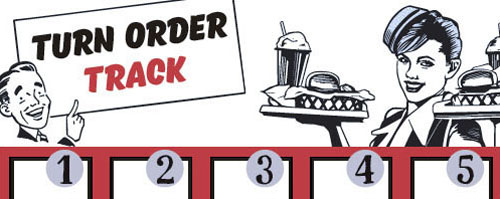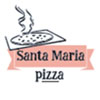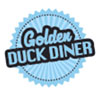## Other Setup

Bank starts with \$200.00
Remove these Billboards: #16
Number of 1x Employee cards used = 2

## Map (Random)

Click here to view Map Tile Lettering Key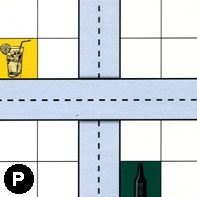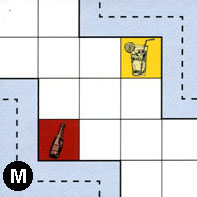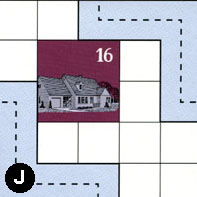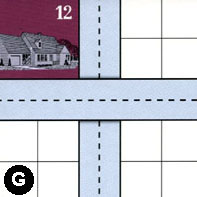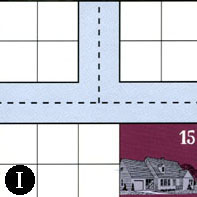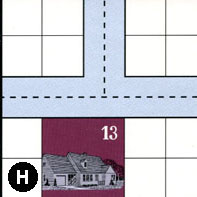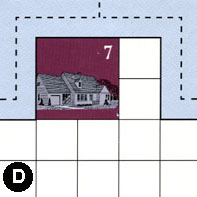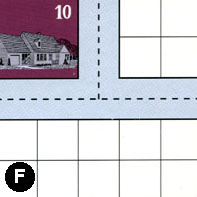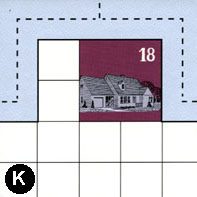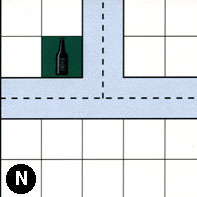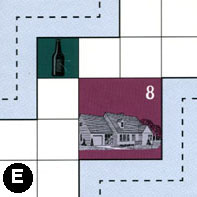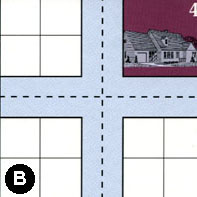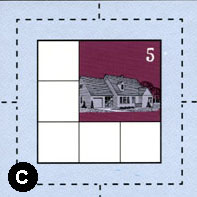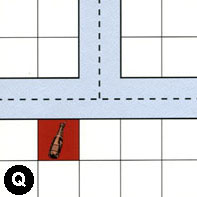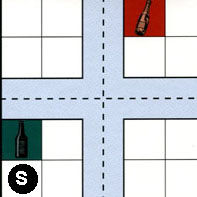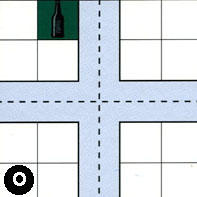### Map Stats

Map Option = Random
Total Number of Tiles = 16
Total Number of Starting Houses = 10
Total Number of Beer Spots = 5
Total Number of Soda Spots = 3
Total Number of Lemonade Spots = 2
Total Number of Drink Spots = 10
Number of independent path systems = 5
Number of independent neighborhoods = 14

Path System 1
Size = Medium
Number of Tiles = 12
Number of Paths = 40
Contains Loop(s) = No
Number of Starting Houses = 8
Starting Houses Pct of Map (%) = 80
Number of Beer Spots = 4
Number of Soda Spots = 2
Number of Lemonade Spots = 1
Number of Drink Spots = 7
Drink Spots Pct of Map (%) = 70
Tile Ids = B, D, E, F, G, H, I, N, O, P, Q, S
Paths = PWE, IWN, IWE, HWE, DWE, FWN, BWN, BNE, OWS, OWN, ONE, SWE, QWN, NWE, HWN, DNE, EES, BWS, FWE, GNS, FNE, DWN, BWE, ONS, OES, SNE, EWN, NWN, HNE, INE, NNE, QWE, QNE, SWN, SNS, SWS, SES, OWE, BES, BNS What do these mean?
Starting House Ids = 0, 4, 7, 8, 10, 12, 13, 15
Path System 2
Size = Small
Number of Tiles = 2
Number of Paths = 2
Contains Loop(s) = No
Number of Starting Houses = 2
Starting Houses Pct of Map (%) = 20
Number of Beer Spots = 1
Number of Soda Spots = 1
Number of Lemonade Spots = 0
Number of Drink Spots = 2
Drink Spots Pct of Map (%) = 20
Tile Ids = M, P
Paths = PNS, MWS What do these mean?
Starting House Ids = 0, 13
Path System 3
Size = Small
Number of Tiles = 2
Number of Paths = 2
Contains Loop(s) = No
Number of Starting Houses = 2
Starting Houses Pct of Map (%) = 20
Number of Beer Spots = 0
Number of Soda Spots = 0
Number of Lemonade Spots = 1
Number of Drink Spots = 1
Drink Spots Pct of Map (%) = 10
Tile Ids = J, M
Paths = MEN, JWS What do these mean?
Starting House Ids = 0, 16
Path System 4
Size = Small
Number of Tiles = 2
Number of Paths = 2
Contains Loop(s) = No
Number of Starting Houses = 2
Starting Houses Pct of Map (%) = 20
Number of Beer Spots = 0
Number of Soda Spots = 0
Number of Lemonade Spots = 0
Number of Drink Spots = 0
Drink Spots Pct of Map (%) = 0
Tile Ids = G, J
Paths = GWE, JEN What do these mean?
Starting House Ids = 12, 16
Path System 5
Size = Medium
Number of Tiles = 2
Number of Paths = 9
Contains Loop(s) = No
Number of Starting Houses = 2
Starting Houses Pct of Map (%) = 20
Number of Beer Spots = 0
Number of Soda Spots = 0
Number of Lemonade Spots = 0
Number of Drink Spots = 0
Drink Spots Pct of Map (%) = 0
Tile Ids = C, K
Paths = CWN, CWE, CNE, CNS, KWE, KWN, KNE, CWS, CES What do these mean?
Starting House Ids = 5, 18

Neighborhood 1
Total Size = Small
Number of Total Spaces = 4
Number of Empty Spaces (for new houses & gardens) = 4
Number of Beer Spots = 0
Number of Soda Spots = 0
Number of Lemonade Spots = 0
Number of Drink Spots = 0
Number of Starting Houses = 0
Starting Houses Pct of Map (%) = 0
Tile Ids = P
Neighborhood 2
Total Size = Large
Number of Total Spaces = 80
Number of Empty Spaces (for new houses & gardens) = 65
Number of Beer Spots = 1
Number of Soda Spots = 1
Number of Lemonade Spots = 1
Number of Drink Spots = 3
Number of Starting Houses = 3
Starting Houses Pct of Map (%) = 30
Tile Ids = D, F, G, H, I, J, M, P
Starting House Ids = 7, 13, 16
Neighborhood 3
Total Size = Small
Number of Total Spaces = 4
Number of Empty Spaces (for new houses & gardens) = 4
Number of Beer Spots = 0
Number of Soda Spots = 0
Number of Lemonade Spots = 0
Number of Drink Spots = 0
Number of Starting Houses = 0
Starting Houses Pct of Map (%) = 0
Tile Ids = G
Neighborhood 4
Total Size = Medium
Number of Total Spaces = 8
Number of Empty Spaces (for new houses & gardens) = 7
Number of Beer Spots = 0
Number of Soda Spots = 0
Number of Lemonade Spots = 1
Number of Drink Spots = 1
Number of Starting Houses = 0
Starting Houses Pct of Map (%) = 0
Tile Ids = I, P
Neighborhood 5
Total Size = Medium
Number of Total Spaces = 18
Number of Empty Spaces (for new houses & gardens) = 14
Number of Beer Spots = 0
Number of Soda Spots = 0
Number of Lemonade Spots = 0
Number of Drink Spots = 0
Number of Starting Houses = 1
Starting Houses Pct of Map (%) = 10
Tile Ids = B, F, G
Starting House Ids = 12
Neighborhood 6
Total Size = Large
Number of Total Spaces = 50
Number of Empty Spaces (for new houses & gardens) = 41
Number of Beer Spots = 0
Number of Soda Spots = 1
Number of Lemonade Spots = 0
Number of Drink Spots = 1
Number of Starting Houses = 2
Starting Houses Pct of Map (%) = 20
Tile Ids = H, I, K, N, Q
Starting House Ids = 15, 18
Neighborhood 7
Total Size = Large
Number of Total Spaces = 35
Number of Empty Spaces (for new houses & gardens) = 28
Number of Beer Spots = 2
Number of Soda Spots = 1
Number of Lemonade Spots = 0
Number of Drink Spots = 3
Number of Starting Houses = 1
Starting Houses Pct of Map (%) = 10
Tile Ids = B, E, H, N, O, S
Starting House Ids = 8
Neighborhood 8
Total Size = Medium
Number of Total Spaces = 8
Number of Empty Spaces (for new houses & gardens) = 4
Number of Beer Spots = 0
Number of Soda Spots = 0
Number of Lemonade Spots = 0
Number of Drink Spots = 0
Number of Starting Houses = 1
Starting Houses Pct of Map (%) = 10
Tile Ids = B, F
Starting House Ids = 10
Neighborhood 9
Total Size = Medium
Number of Total Spaces = 12
Number of Empty Spaces (for new houses & gardens) = 12
Number of Beer Spots = 0
Number of Soda Spots = 0
Number of Lemonade Spots = 0
Number of Drink Spots = 0
Number of Starting Houses = 0
Starting Houses Pct of Map (%) = 0
Tile Ids = N, Q, S
Neighborhood 10
Total Size = Medium
Number of Total Spaces = 8
Number of Empty Spaces (for new houses & gardens) = 4
Number of Beer Spots = 0
Number of Soda Spots = 0
Number of Lemonade Spots = 0
Number of Drink Spots = 0
Number of Starting Houses = 1
Starting Houses Pct of Map (%) = 10
Tile Ids = B, O
Starting House Ids = 4
Neighborhood 11
Total Size = Medium
Number of Total Spaces = 9
Number of Empty Spaces (for new houses & gardens) = 5
Number of Beer Spots = 0
Number of Soda Spots = 0
Number of Lemonade Spots = 0
Number of Drink Spots = 0
Number of Starting Houses = 1
Starting Houses Pct of Map (%) = 10
Tile Ids = C
Starting House Ids = 5
Neighborhood 12
Total Size = Medium
Number of Total Spaces = 8
Number of Empty Spaces (for new houses & gardens) = 7
Number of Beer Spots = 1
Number of Soda Spots = 0
Number of Lemonade Spots = 0
Number of Drink Spots = 1
Number of Starting Houses = 0
Starting Houses Pct of Map (%) = 0
Tile Ids = Q, S
Neighborhood 13
Total Size = Medium
Number of Total Spaces = 8
Number of Empty Spaces (for new houses & gardens) = 8
Number of Beer Spots = 0
Number of Soda Spots = 0
Number of Lemonade Spots = 0
Number of Drink Spots = 0
Number of Starting Houses = 0
Starting Houses Pct of Map (%) = 0
Tile Ids = O, S
Neighborhood 14
Total Size = Small
Number of Total Spaces = 4
Number of Empty Spaces (for new houses & gardens) = 3
Number of Beer Spots = 1
Number of Soda Spots = 0
Number of Lemonade Spots = 0
Number of Drink Spots = 1
Number of Starting Houses = 0
Starting Houses Pct of Map (%) = 0
Tile Ids = O

v2.1.5